# EEG source localization given electrode locations on an MRI¶

This tutorial explains how to compute the forward operator from EEG data when the electrodes are in MRI voxel coordinates.

```# Authors: Eric Larson <larson.eric.d@gmail.com>
#

import os.path as op

import nibabel
from nilearn.plotting import plot_glass_brain
import numpy as np

import mne
from mne.viz import plot_alignment
```

## Prerequisites¶

For this we will assume that you have:

• raw EEG data

• your subject’s MRI reconstrcted using FreeSurfer

• an appropriate boundary element model (BEM)

• an appropriate source space (src)

• your EEG electrodes in Freesurfer surface RAS coordinates, stored in one of the formats `mne.channels.read_custom_montage()` supports

Let’s set the paths to these files for the `sample` dataset, including a modified `sample` MRI showing the electrode locations plus a `.elc` file corresponding to the points in MRI coords (these were synthesized, and thus are stored as part of the `misc` dataset).

```data_path = mne.datasets.sample.data_path()
subjects_dir = op.join(data_path, 'subjects')
fname_raw = op.join(data_path, 'MEG', 'sample', 'sample_audvis_raw.fif')
bem_dir = op.join(subjects_dir, 'sample', 'bem')
fname_bem = op.join(bem_dir, 'sample-5120-5120-5120-bem-sol.fif')
fname_src = op.join(bem_dir, 'sample-oct-6-src.fif')

misc_path = mne.datasets.misc.data_path()
fname_T1_electrodes = op.join(misc_path, 'sample_eeg_mri', 'T1_electrodes.mgz')
fname_mon = op.join(misc_path, 'sample_eeg_mri', 'sample_mri_montage.elc')
```

## Visualizing the MRI¶

Let’s take our MRI-with-eeg-locations and adjust the affine to put the data in MNI space, and plot using `nilearn.plotting.plot_glass_brain()`, which does a maximum intensity projection (easy to see the fake electrodes). This plotting function requires data to be in MNI space. Because `img.affine` gives the voxel-to-world (RAS) mapping, if we apply a RAS-to-MNI transform to it, it becomes the voxel-to-MNI transformation we need. Thus we create a “new” MRI image in MNI coordinates and plot it as:

```img = nibabel.load(fname_T1_electrodes)  # original subject MRI w/EEG
ras_mni_t = mne.transforms.read_ras_mni_t('sample', subjects_dir)  # from FS
mni_affine = np.dot(ras_mni_t['trans'], img.affine)  # vox->ras->MNI
img_mni = nibabel.Nifti1Image(img.dataobj, mni_affine)  # now in MNI coords!
plot_glass_brain(img_mni, cmap='hot_black_bone', threshold=0., black_bg=True,
resampling_interpolation='nearest', colorbar=True)
```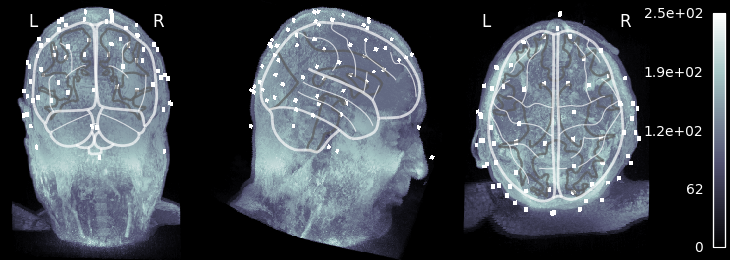## Getting our MRI voxel EEG locations to head (and MRI surface RAS) coords¶

Let’s load our `DigMontage` using `mne.channels.read_custom_montage()`, making note of the fact that we stored our locations in Freesurfer surface RAS (MRI) coordinates.

#### What if my electrodes are in MRI voxels?

If you have voxel coordinates in MRI voxels, you can transform these to FreeSurfer surface RAS (called “mri” in MNE) coordinates using the transformations that FreeSurfer computes during reconstruction. `nibabel` calls this transformation the `vox2ras_tkr` transform and operates in millimeters, so we can load it, convert it to meters, and then apply it:

```>>> pos_vox = ...  # loaded from a file somehow
>>> vox2mri_t = img.header.get_vox2ras_tkr()  # voxel -> mri trans
>>> pos_mri = mne.transforms.apply_trans(vox2mri_t, pos_vox)
>>> pos_mri /= 1000.  # mm -> m
```

You can also verify that these are correct (or manually convert voxels to MRI coords) by looking at the points in Freeview or tkmedit.

```dig_montage = read_custom_montage(fname_mon, head_size=None, coord_frame='mri')
dig_montage.plot()
```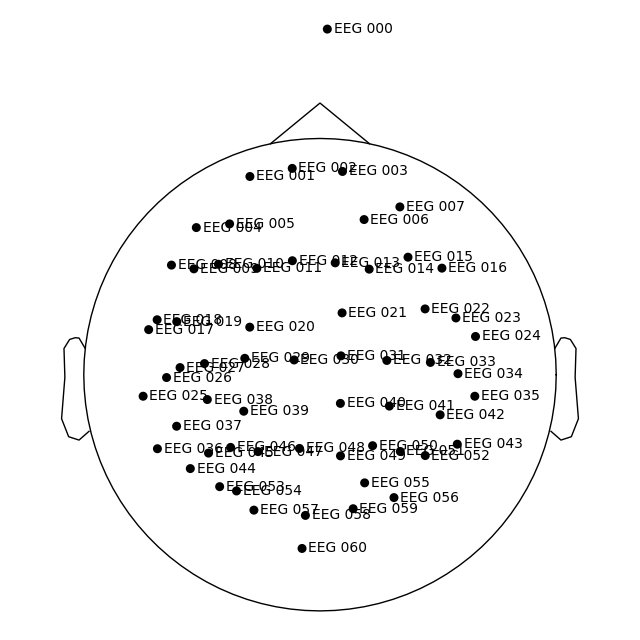Out:

```Creating RawArray with float64 data, n_channels=61, n_times=1
Range : 0 ... 0 =      0.000 ...     0.000 secs
```

We can then get our transformation from the MRI coordinate frame (where our points are defined) to the head coordinate frame from the object.

```trans = compute_native_head_t(dig_montage)
print(trans)  # should be mri->head, as the "native" space here is MRI
```

Out:

```<Transform | MRI (surface RAS)->head>
[[ 0.99930957  0.00998471 -0.03578661 -0.00316747]
[ 0.01275917  0.81240435  0.58295487  0.00685511]
[ 0.03489383 -0.58300899  0.81171605  0.02888406]
[ 0.          0.          0.          1.        ]]
```

Let’s apply this digitization to our dataset, and in the process automatically convert our locations to the head coordinate frame, as shown by `plot_sensors()`.

```raw = mne.io.read_raw_fif(fname_raw)
raw.set_montage(dig_montage)
raw.plot_sensors(show_names=True)
```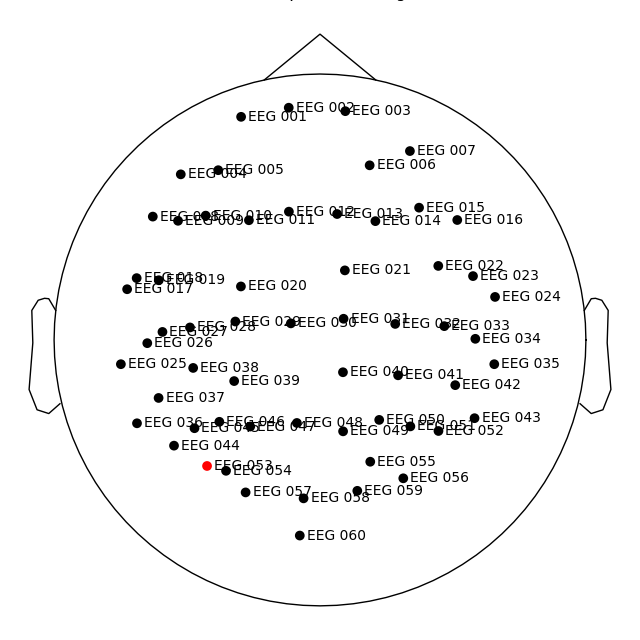Out:

```Opening raw data file /home/circleci/mne_data/MNE-sample-data/MEG/sample/sample_audvis_raw.fif...
Read a total of 3 projection items:
PCA-v1 (1 x 102)  idle
PCA-v2 (1 x 102)  idle
PCA-v3 (1 x 102)  idle
Range : 25800 ... 192599 =     42.956 ...   320.670 secs
Removing projector <Projection | PCA-v1, active : False, n_channels : 102>
Removing projector <Projection | PCA-v2, active : False, n_channels : 102>
Removing projector <Projection | PCA-v3, active : False, n_channels : 102>
Reading 0 ... 166799  =      0.000 ...   277.714 secs...
```

Now we can do standard sensor-space operations like make joint plots of evoked data.

```raw.set_eeg_reference(projection=True)
events = mne.find_events(raw)
epochs = mne.Epochs(raw, events)
cov = mne.compute_covariance(epochs, tmax=0.)
evoked = epochs['1'].average()  # trigger 1 in auditory/left
evoked.plot_joint()
```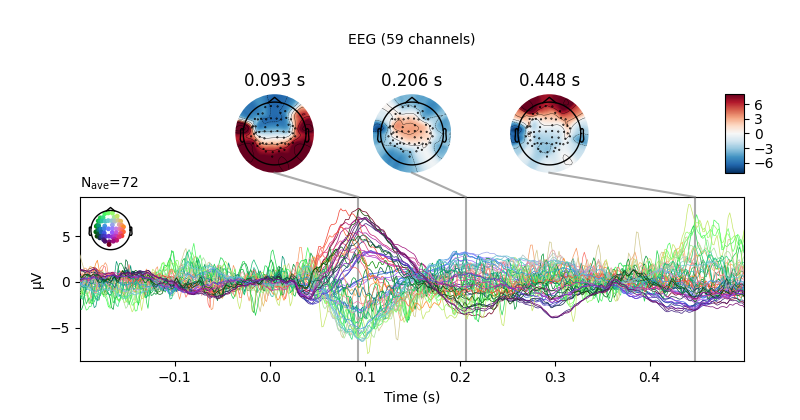Out:

```Adding average EEG reference projection.
1 projection items deactivated
Average reference projection was added, but has not been applied yet. Use the apply_proj method to apply it.
320 events found
Event IDs: [ 1  2  3  4  5 32]
320 matching events found
Setting baseline interval to [-0.19979521315838786, 0.0] sec
Applying baseline correction (mode: mean)
Created an SSP operator (subspace dimension = 1)
1 projection items activated
Computing rank from data with rank=None
Using tolerance 7.7e-11 (2.2e-16 eps * 59 dim * 5.9e+03  max singular value)
Estimated rank (eeg): 58
EEG: rank 58 computed from 59 data channels with 1 projector
Created an SSP operator (subspace dimension = 1)
Setting small EEG eigenvalues to zero (without PCA)
Reducing data rank from 59 -> 58
Estimating covariance using EMPIRICAL
Done.
Number of samples used : 38720
[done]
Projections have already been applied. Setting proj attribute to True.
```

## Getting a source estimate¶

New we have all of the components we need to compute a forward solution, but first we should sanity check that everything is well aligned:

```fig = plot_alignment(
subject='sample', subjects_dir=subjects_dir)
```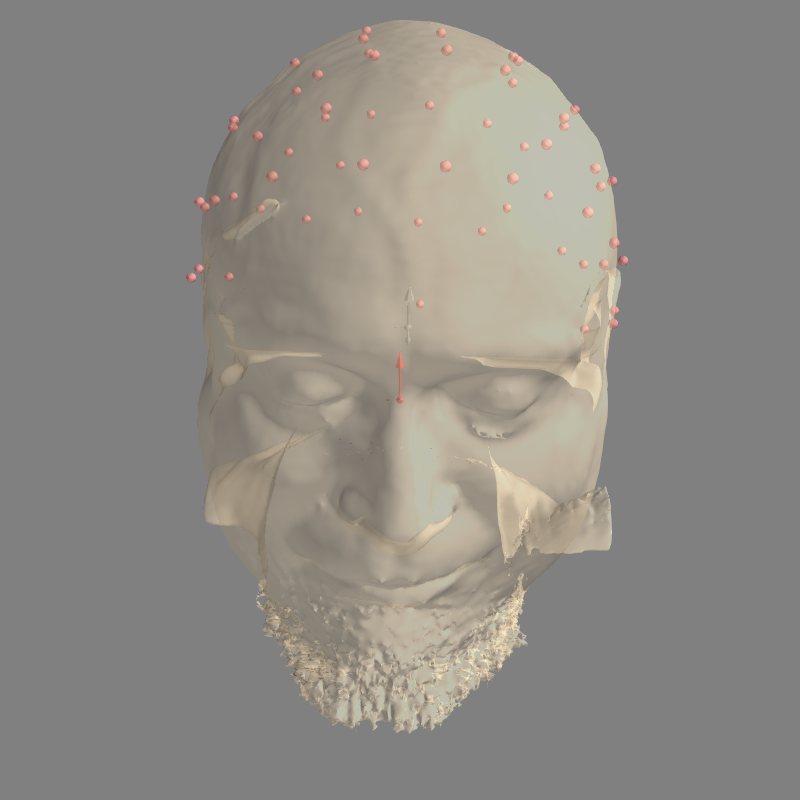Out:

```Using lh.seghead for head surface.
```

Now we can actually compute the forward:

```fwd = mne.make_forward_solution(
evoked.info, trans=trans, src=fname_src, bem=fname_bem, verbose=True)
```

Out:

```Source space          : /home/circleci/mne_data/MNE-sample-data/subjects/sample/bem/sample-oct-6-src.fif
MRI -> head transform : instance of Transform
Measurement data      : instance of Info
Conductor model   : /home/circleci/mne_data/MNE-sample-data/subjects/sample/bem/sample-5120-5120-5120-bem-sol.fif
Accurate field computations
Free source orientations

Read 2 source spaces a total of 8196 active source locations

Coordinate transformation: MRI (surface RAS) -> head
0.999310  0.009985 -0.035787      -3.17 mm
0.012759  0.812404  0.582955       6.86 mm
0.034894 -0.583009  0.811716      28.88 mm
0.000000  0.000000  0.000000       1.00

Read  60 EEG channels from info
Source spaces are now in head coordinates.

Setting up the BEM model using /home/circleci/mne_data/MNE-sample-data/subjects/sample/bem/sample-5120-5120-5120-bem-sol.fif...

Loaded linear_collocation BEM solution from /home/circleci/mne_data/MNE-sample-data/subjects/sample/bem/sample-5120-5120-5120-bem-sol.fif
Employing the head->MRI coordinate transform with the BEM model.
BEM model sample-5120-5120-5120-bem-sol.fif is now set up

Source spaces are in head coordinates.
Checking that the sources are inside the surface (will take a few...)
Skipping interior check for 846 sources that fit inside a sphere of radius   43.6 mm
Skipping solid angle check for 2 points using Qhull
2 source space points omitted because they are outside the inner skull surface.
Computing patch statistics...
Skipping interior check for 875 sources that fit inside a sphere of radius   43.6 mm
Skipping solid angle check for 0 points using Qhull
1 source space point omitted because it is outside the inner skull surface.
Computing patch statistics...

Setting up for EEG...
Computing EEG at 8193 source locations (free orientations)...

Finished.
```

Finally let’s compute the inverse and apply it:

```inv = mne.minimum_norm.make_inverse_operator(
evoked.info, fwd, cov, verbose=True)
stc = mne.minimum_norm.apply_inverse(evoked, inv)
brain = stc.plot(subjects_dir=subjects_dir, initial_time=0.1)
```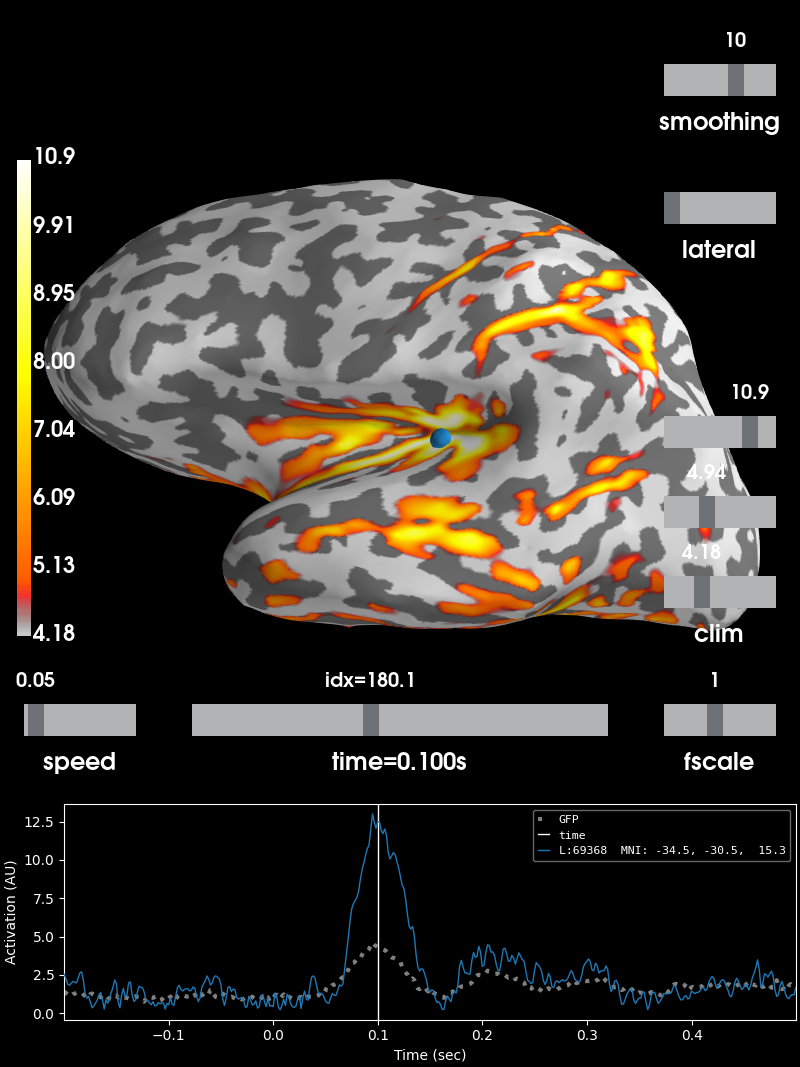Out:

```Converting forward solution to surface orientation
Average patch normals will be employed in the rotation to the local surface coordinates....
Converting to surface-based source orientations...
[done]
Computing inverse operator with 59 channels.
59 out of 60 channels remain after picking
Selected 59 channels
Creating the depth weighting matrix...
59 EEG channels
limit = 8192/8193 = 10.040598
scale = 157084 exp = 0.8
Applying loose dipole orientations to surface source spaces: 0.2
Whitening the forward solution.
Created an SSP operator (subspace dimension = 1)
Computing rank from covariance with rank=None
Using tolerance 1.2e-13 (2.2e-16 eps * 59 dim * 9  max singular value)
Estimated rank (eeg): 58
EEG: rank 58 computed from 59 data channels with 1 projector
Setting small EEG eigenvalues to zero (without PCA)
Creating the source covariance matrix
Computing SVD of whitened and weighted lead field matrix.
largest singular value = 2.86184
scaling factor to adjust the trace = 3.24877e+19
Preparing the inverse operator for use...
Scaled noise and source covariance from nave = 1 to nave = 72
Created the regularized inverter
Created an SSP operator (subspace dimension = 1)
Created the whitener using a noise covariance matrix with rank 58 (1 small eigenvalues omitted)
Computing noise-normalization factors (dSPM)...
[done]
Applying inverse operator to "1"...
Picked 59 channels from the data
Computing inverse...
Eigenleads need to be weighted ...
Computing residual...
Explained  85.0% variance
Combining the current components...
dSPM...
[done]
Using control points [ 4.17591891  4.939572   10.86348066]
```

Total running time of the script: ( 0 minutes 52.335 seconds)

Estimated memory usage: 465 MB

Gallery generated by Sphinx-Gallery